# Tutorials

## Visualization of structural variation with VCF

It is possible to visualize structural variation (SV) using VCF data (`vcf_sv.py`):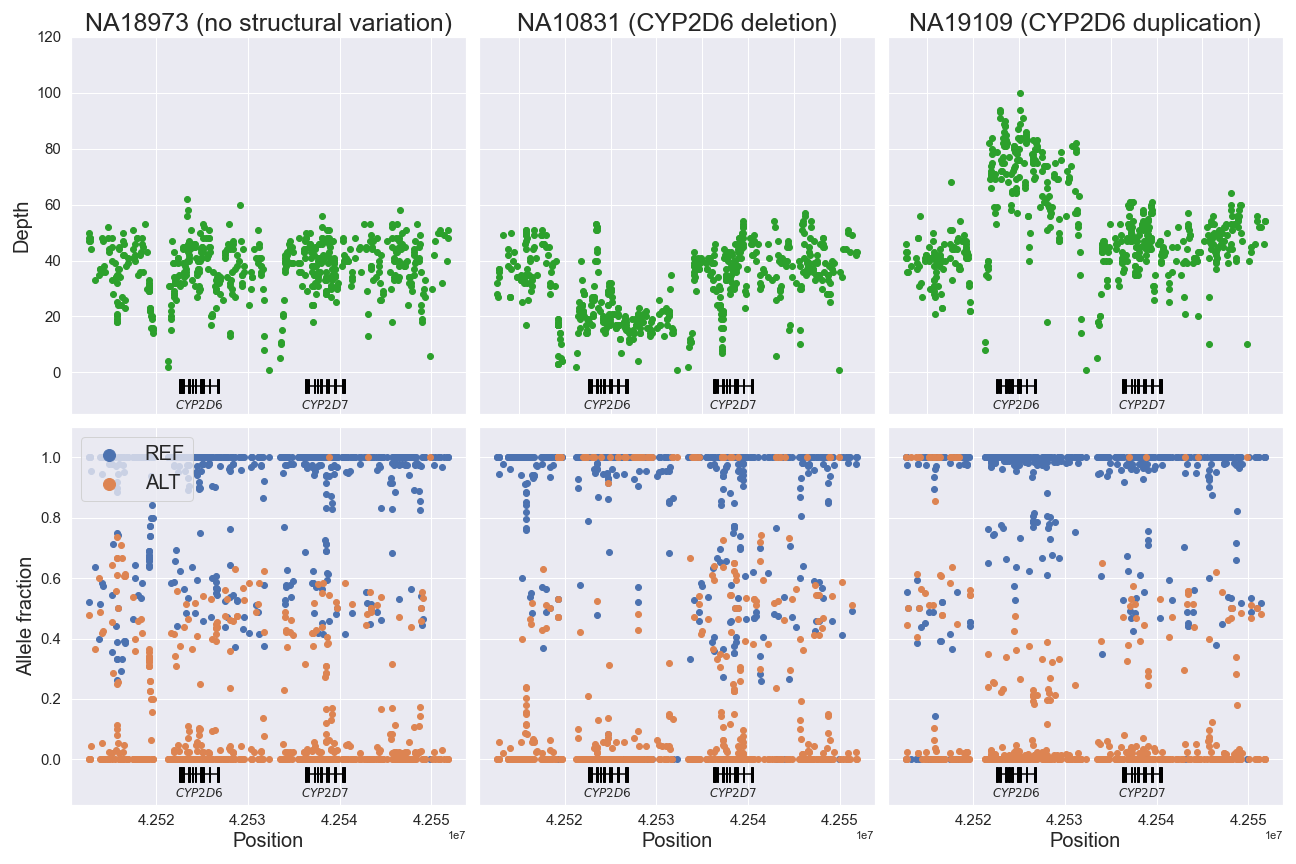## Create customized oncoplots

We can use either the maf_oncoplt command or the `pymaf.plot_oncoplot()` method to create a “standard” oncoplot like the one shown below.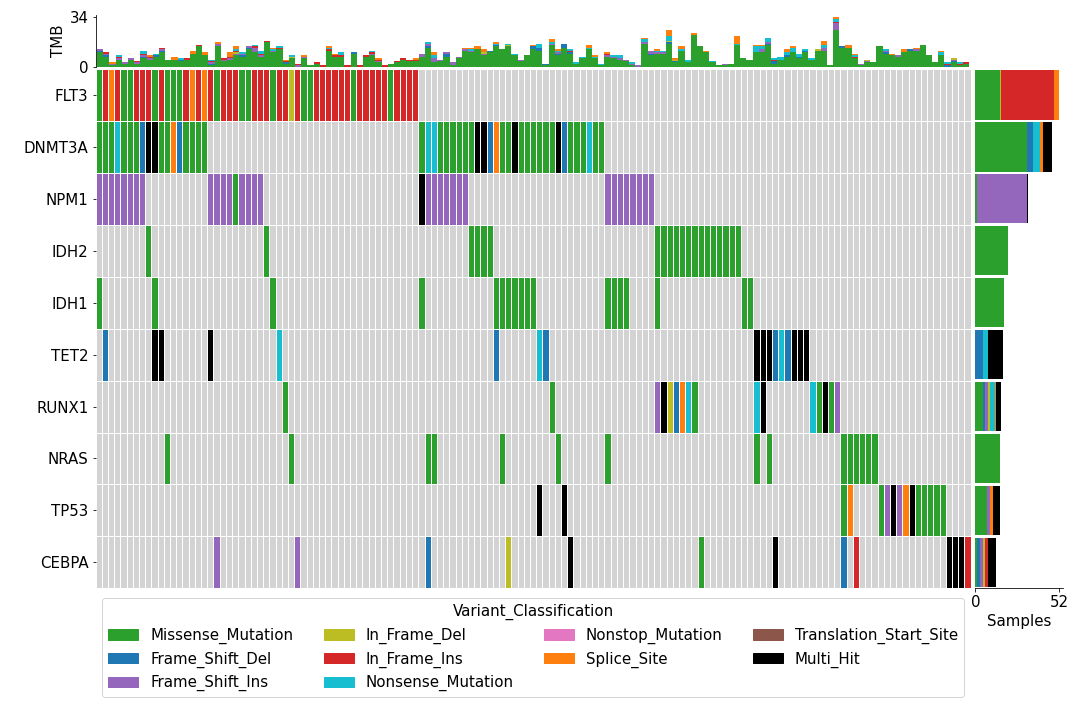While the plot is pleasing to the eye, one may wish to customize it to add, for example, various annotation data for the samples like this: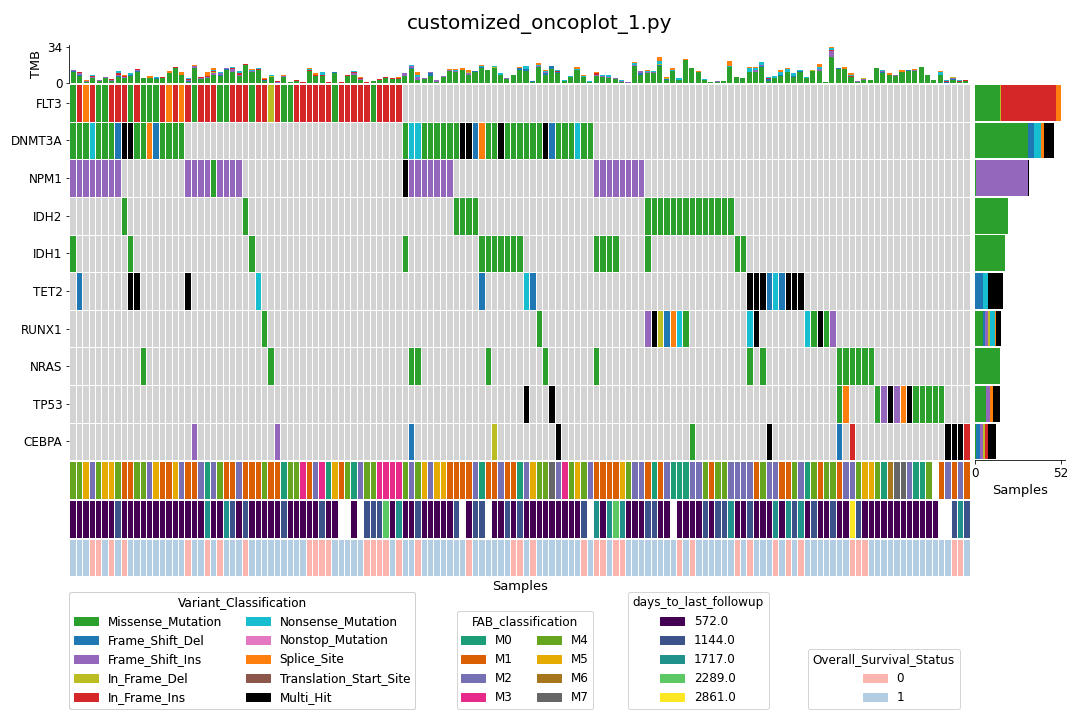We can (relatively) easily achieve above thanks to the LEGO block-like plotting methods in the `pymaf` submodule (`customized_oncoplot_1.py`).

We can go one step further and sort the samples by one or more annotations (`customized_oncoplot_2.py`):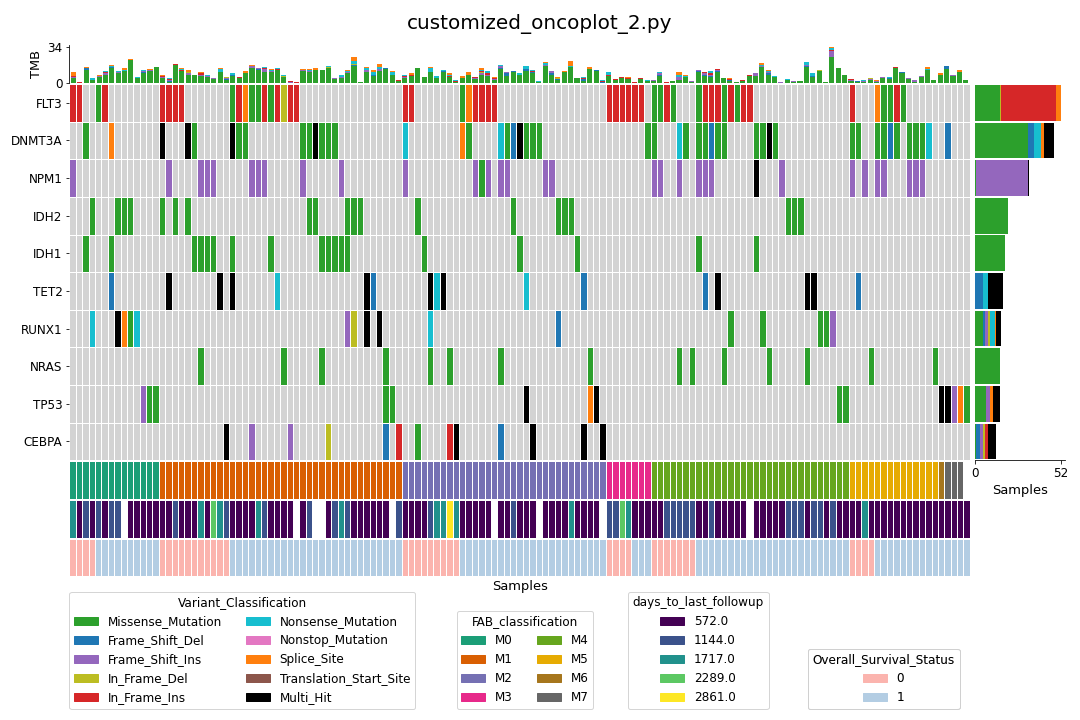Finally, we can also subset the samples with annotation data (`customized_oncoplot_3.py`):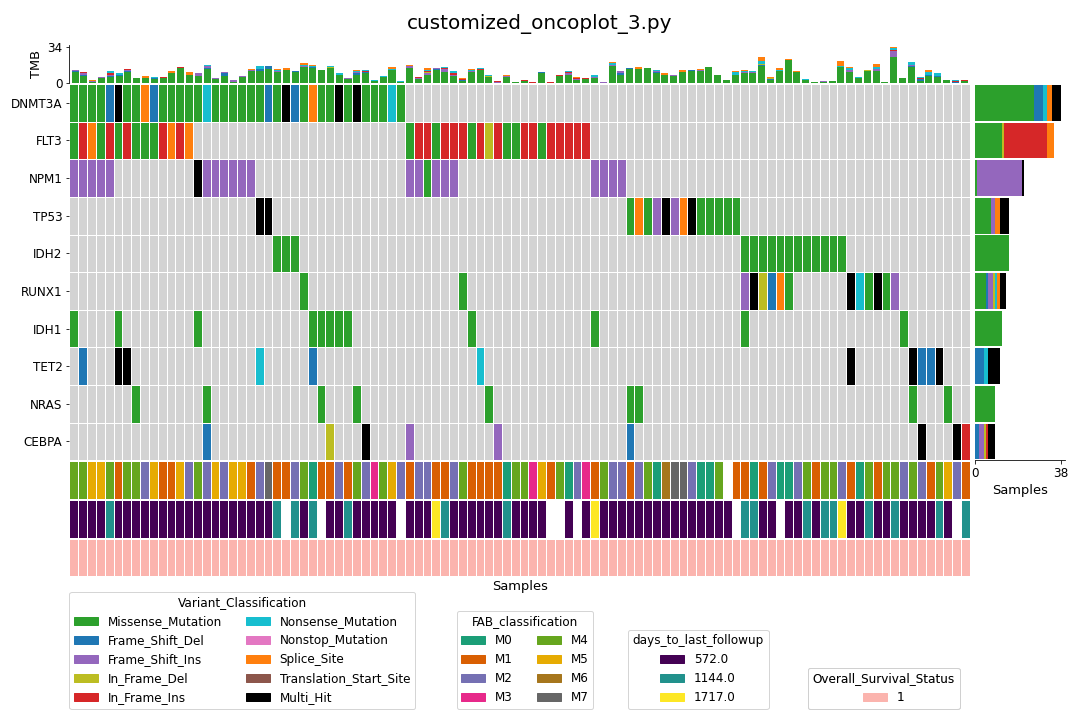## Control plot colors

Let us consider the `fuc.api.pymaf.MafFrame.plot_snvclsc()` method as an example. The method internally uses `seaborn.barplot()` to draw bar plots.

```from fuc import common, pymaf
maf_file = '~/fuc-data/tcga-laml/tcga_laml.maf.gz'
mf = pymaf.MafFrame.from_file(maf_file)
mf.plot_snvclsc()
```

In order to change the colors according to one of the seaborn palettes (e.g. ‘deep’, ‘muted’, ‘pastel’), we can use the `palette` option:

```import seaborn as sns
mf.plot_snvclsc(palette='pastel')
```

To choose one of the colormaps available in maplotlib (e.g. ‘tab10’, ‘Dark2’, ‘Pastel1’), we can use the `palette` option with `seaborn.color_palette()`:

```mf.plot_snvclsc(palette=sns.color_palette('Pastel1'))
```

Some plotting methods use maplotlib instead of seaborn for coloring, like the `fuc.api.pymaf.MafFrame.plot_snvclss()` method:

```ax = mf.plot_snvclss(width=1)
ax.legend(loc='upper right')
```

To choose a colormap from maplotlib:

```import matplotlib.pyplot as plt
ax = mf.plot_snvclss(width=1, color=plt.get_cmap('Pastel1').colors)
ax.legend(loc='upper right')
```

To choose a palette from seaborn:

```ax = mf.plot_snvclss(width=1, color=sns.color_palette('pastel'))
ax.legend(loc='upper right')
```